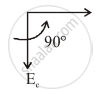Share

# If A.C. Voltage is Applied to a Pure Capacitor, Then Voltage Across the Capacitor - Physics

#### Question

If A.C. voltage is applied to a pure capacitor, then voltage across the capacitor _____________.

##### Options

• lags behind the current by phase angle (π/2) rad.

• lags behind the current by phase angle π rad.

#### Solution

lags behind the current by phase angle (π/2) rad.Is there an error in this question or solution?